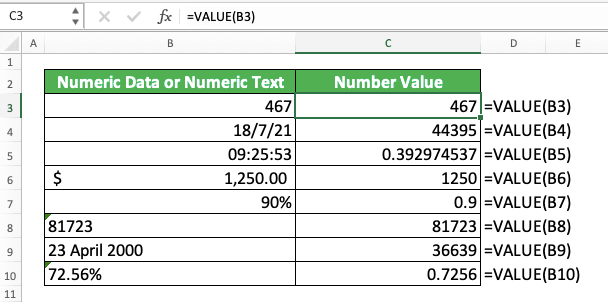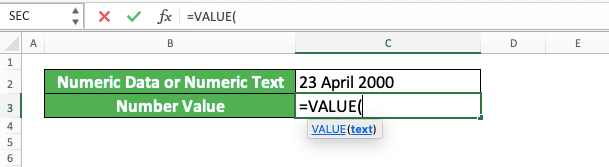How to Use the VALUE Formula in Excel: Functions, Examples, and Writing Steps - Compute Expert

# How to Use the VALUE Formula in Excel: Functions, Examples, and Writing Steps

Home >> Excel Tutorials from Compute Expert >> Excel Formulas List >> How to Use the VALUE Formula in Excel: Functions, Examples, and Writing Steps

In this tutorial, you will learn how to use the VALUE formula in excel completely.

When working in excel, we may sometimes need to convert a number text or numeric data (e.g. date, time, a number with a currency symbol) into its pure numeric value. If we know how to use VALUE in excel, then we can get that value fast and easily.

Want to know more about VALUE and master the way to use it in excel? Read this tutorial until its last part!

Disclaimer: This post may contain affiliate links from which we earn commission from qualifying purchases/actions at no additional cost for you. Learn more

## What is the VALUE Formula in Excel?

VALUE is an excel function that helps you to get the pure value of numeric data or text in excel.

## VALUE Function in Excel

You can use VALUE to get the pure number value of a numeric data or text you input into it.

## VALUE Result

The VALUE result is a number that represents the value of the numeric data or text you input into it.

## Excel Version from Which We Can Start Using VALUE

We can start using VALUE in excel since excel 2003.

## The Way to Write It and Its Input

Here is the way to write VALUE in excel.

= VALUE ( numeric_data_or_numeric_text )

You just need to input the numeric data or the numeric text you want to get the pure value from. Note that what numeric text means here is a text which contains data excel recognizes as numeric data.

## Example of Its Usage and Result

Here is an implementation example of VALUE in excel.As you can see in the example, VALUE can get the pure number value of your numeric data or text. Just input your numeric data or text into VALUE, press enter, and you will immediately get its number value!

## Writing Steps

Need guidance when you write your VALUE formula in excel? Use these writing steps we have made for you below!

1. Type an equal sign ( = ) in the cell where you want to put your VALUE result2. Type VALUE (can be with large letters and small letters) and an open bracket sign after =3. Input the numeric data or numeric text you want to get your number value from4. Type a close bracket sign5. Press Enter
6. Done!## Exercise

After you have learned how to use VALUE in excel, let’s do the following exercise to deepen your understanding!

### Questions

Give your answer in the appropriate gray-colored cell according to the question number. Use VALUE to get the answer.
1. What is the number value of the time data on the left?
2. What is the sum of the number on the left with 2? Use SUM to sum the two numbers!
3. What is the average of the number on the left with the no. 2 number? Use AVERAGE to get the average of the two numbers!

Confused about the VALUE result you get from a date/time? That is the serial number for that date/time in excel.

In excel, date/time data is actually a number that it displays differently. That number before excel changes its display is what VALUE produces for you.

Related tutorials you should learn too:

Get updated excel info from Compute Expert by registering your email. It's free!